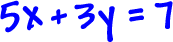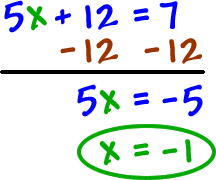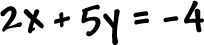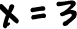We just need to figure out what the x is.  Substitution!  Take the y guy and stick it into the first equation:This gives.  Solve for x!Let's double-check that:So, the solution to our system is.

Remember that this is a point where two lines intersect.

TRY IT:

Solve by substitution: